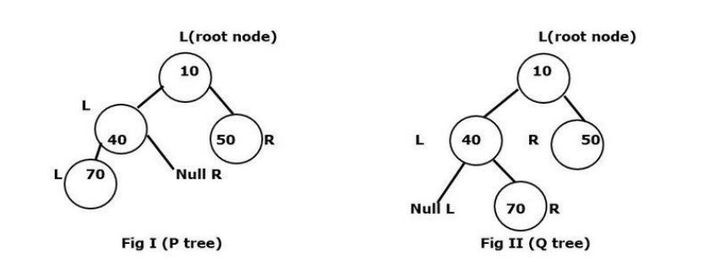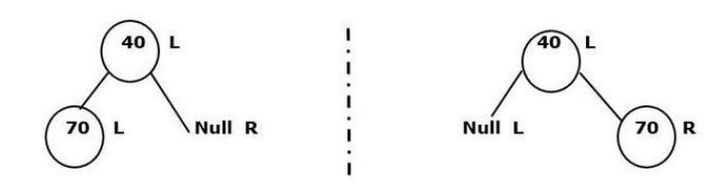# Isomorphism in N-ary Trees

Isomorphism is defined as two trees either having identical or mirror structures. In the case of mirror structure, the left node data will always match the right node. For example, we will take a number nearest to the mirror and see what its reverse would be, that is the real concept of isomorphism.

In this article, we are going to check whether two different binary trees are isomorphic or not.

Let’s take an example of Isomorphism in N-ary Trees −Note that, L represents as left node whereas R represents as Right node

Mirror structure of P and Q tree on leftmost second partitioningThese two figures show how they are isomorphic to each other by giving four matching conditions (root node of P and Q) such as

• Either the left-left node can be matched.

• Either the right-right node can be matched.

• Either the left-right node can be matched.

• Either the right-left no can be matched.

## Syntax

The following syntax used in the program −

struct name_of_structure{
data_type var_name;
// data member or field of the structure.
}


## Parameters

• struct − This keyword is used to represent structure datatype.

• name_of_structure − We are providing any name to the structure.

• The structure is a collection of various related variables in one place.

## Algorithm

• We will start the program with a header file named ‘iostream’.

• We are creating the structure named ‘tree_node’ with integer type ‘d’ and initialize the pointer variables- ‘l’ and ‘r’ to represent the left and right child nodes data respectively.

• Now we create another structure with a function named ‘create_node()’ which accepts a parameter named ‘data’ to address the value for the root node. Also, we create the ‘tree_node’ pointer with the given data to initialize the left and right child pointer to null and return the root node. Using this function, we will insert the nodes of both left and right child.

• We are creating function ‘check_isomorphism_tree with bool datatype to take two tree_node pointers p and q as input parameters and return a boolean value. Inside this, we create an "if-statement" twice to check whether the data in p is equivalent to q or not.

• Check if both p andq are null, if yes then return true as the trees are isomorphic.

• Check if any of p or q is null, if yes then return false as the trees are not isomorphic.

• In the ‘check_isomorphism_tree’ function, we recursively check all possible combinations of left and right child of node ‘p’ and ‘q’ using the logical operator “&&” and “||”.

• We start with the main function and create two tree nodes ‘p’ and ‘q’ to provide the information.

• In the main function, we use the if-statement to call the ‘check_isomorphism_tree’ function and pass the given parameters p and q to verify whether these integer values are isomorphic. If it is isomorphic then print the statement as “This given information of node will make isomorphism tree” otherwise vice versa.

## Example

In this program, we are going to check whether two binary trees are isomorphic or not.

#include<iostream>
using namespace std;
struct tree_node{
int d;
tree_node*l;  // l = left
tree_node*r;  // r = right
};
struct tree_node* create_node(int data){
struct tree_node*root= new tree_node;
root->d= data;
root->l= NULL;
root->r= NULL;
return root;
}
bool check_isomorphism_tree(tree_node*p, tree_node*q)  {
// p and q both are different tree
if(p==NULL and q==NULL){
return true;
}
if(p==NULL or q==NULL){
return false;
}
// return all the possible condition
return (p->d==q->d && ((check_isomorphism_tree(p->l,q->r)&& check_isomorphism_tree(p->r,q->l))||(check_isomorphism_tree(p->l,q->l)&& check_isomorphism_tree(p->r,q->r))));
}
int main(){
// Tree of root p
struct tree_node *p = create_node(10);
p->l  = create_node(5);
p->r = create_node(4);
p->l->l = create_node(11);
p->r->r = create_node(12);
p->l->r = create_node(51);
p->r->l = create_node(6);
p->l->r->l = create_node(7); // left->right->left
p->l->l->l = create_node(9); // left->left->left
// Tree of root q
struct tree_node *q = create_node(10);
q->l  = create_node(5);
q->r = create_node(4);
q->l->l = create_node(11);
q->r->r = create_node(12);
q->l->r = create_node(51);
q->r->l = create_node(6);
q->l->r->l = create_node(7);
q->l->l->l = create_node(9);
if(check_isomorphism_tree(p,q)){
cout<<"This given information of node will make isomorphism tree"<<endl;
} else {
cout<<" This given information of node will not make isomorphism tree "<<endl;
}
return 0;
}


## Output

This given information of node will make isomorphism tree


## Conclusion

In this program, we understood the concept of Isomorphism in N-ary trees. We saw how a structure helps represent the tree nodes and the use of left-left node, right-left node, left-right-left node, etc. helps to build the trees and the following operation helps to satisfy the tree isomorphism in nature.

Updated on: 20-Apr-2023

253 Views